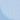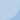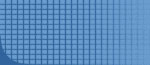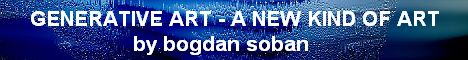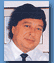Biography
 Home       ABOUT AUTHOR   biography   statement   curriculum   history   art critic GENERATIVE ARTGALERIESDEMO PROGRAMSOTHERCONTACT   contact KONEC LINKI GA konference  GA linki Drugi linki OBISKOVALCI ste obiskovalec  št.:  262987 od okt/2002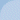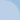CURVED OBJECT 01 - program rotation03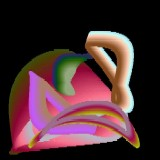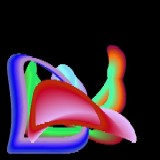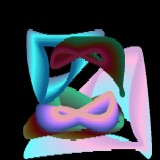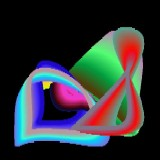Image properties: Picture box Picture1, Cartesian plain: x from –3 to +3; y from –3 to +3; dimension: width 500 pixels, height 500 pixels, command button for next image Result description: Sculpture composed of 5 curved objects, iridescent colors Basic concept: deformed elliptical rotation with random start position and extremely small angle step as the basic point trajectory. On the current point position the program draws a circle colored by random selected basic color for the object with increased values of RGB components. Algorithm description: At first some constants for the current object are defined: r = radius, fi = basic angle, dfi = basic angle step, fx and fy = auxiliary angles, dfxy = auxiliary angle step, re, gr, bl = basic RGB components. Inside the main loop the values of constants increase depending on selected steps. The most important task is to calculate the current point position (x and y), using current values of r, fi, fx, and fy and to transform them into pixel values (i and j). Then the algorithm defines the current RGB color components and finally draws colored circle. The main loop repeats 5 times. Download and run exe version Visual Basic source code Public fre, fgr, fbl, fr Private Sub Command1_Click()      Picture1.Cls: Call draw End Sub Private Sub Form_Load()      Form1.Show: Randomize Timer: Cls      Picture1.BackColor = RGB(0, 0, 0): Picture1.FillStyle = 0      fre = 1: fgr = 1: fbl = 1: fr = 1: Call draw End Sub Private Sub draw()      Randomize Timer: On Error Resume Next      For nn = 1 To 5      r = 1 + Rnd * 1.8: dr = 0.000005: fi = Rnd * 6.286: dfi = (Rnd * 1) / 100000      fx = Int(Rnd * 5): fy = Int(Rnd * 5): dfxy = (2 + Rnd * 7) / 1000      re = Rnd * 256: gr = Rnd * 256: bl = Rnd * 256      dre = (Rnd * 1) / 300: dgr = (Rnd * 1) / 300: dbl = (Rnd * 1) / 300      For n = 1 To 100000      If r < -2.5 Then fr = 1      If r > 2.5 Then fr = -1      r = r + dr * fr: fi = fi + dfi: fx = fx + dfxy: fy = fy + dfxy      x = r * Cos(fi + Sin(fx)): y = r * Sin(fi + Cos(fy))      i = (x + 3) * (500 / 6): j = (3 - y) * (500 / 6)      If re > 255 Then fre = -1      If re < 2 Then fre = 1      If gr > 255 Then fgr = -1      If gr < 2 Then fgr = 1      If bl > 255 Then fbl = -1      If bl < 2 Then fbl = 1      re = re + dre * fre: gr = gr + dgr * fgr: bl = bl + dbl * fbl      Picture1.FillColor = RGB(re, gr, bl)      Picture1.Circle (i, j), 5, RGB(re, gr, bl)      Next n: Next nn End Sub